# 5.5 Potential, Free Energy, and Equilibrium

Learning Objectives

By the end of this section, you will be able to:

• Explain the relations between potential, free energy change, and equilibrium constants
• Perform calculations involving the relations between cell potentials, free energy changes, and equilibrium
• Use the Nernst equation to determine cell potentials under nonstandard conditions

So far in this chapter, the relationship between the cell potential and reaction spontaneity has been described, suggesting a link to the free energy change for the reaction (see chapter on thermodynamics). The interpretation of potentials as measures of oxidant strength was presented, bringing to mind similar measures of acid-base strength as reflected in equilibrium constants (see the chapter on acid-base equilibria). This section provides a summary of the relationships between potential and the related thermodynamic properties ΔG and K.

## E° and ΔG°

The standard free energy change of a process, ΔG°, was defined in a previous chapter as the maximum work that could be performed by a system, wmax. In the case of a redox reaction taking place within a galvanic cell under standard state conditions, essentially all the work is associated with transferring the electrons from reductant-to-oxidant, welec: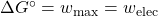The work associated with transferring electrons is determined by the total amount of charge (coulombs) transferred and the cell potential: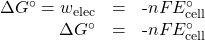where n is the number of moles of electrons transferred, F is Faraday’s constant, and E°cell is the standard cell potential. The relation between free energy change and standard cell potential confirms the sign conventions and spontaneity criteria previously discussed for both of these properties: spontaneous redox reactions exhibit positive potentials and negative free energy changes.

## E° and K

Combining a previously derived relation between ΔG° and K (see the chapter on thermodynamics) and the equation above relating ΔG° and E°cell yields the following: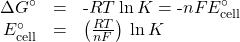This equation indicates redox reactions with large (positive) standard cell potentials will proceed far towards completion, reaching equilibrium when the majority of reactant has been converted to product. A summary of the relations between E°, ΔG° and K is depicted in (Figure 5.5.1), and a table correlating reaction spontaneity to values of these properties is provided in (Table 5.5.1).

Table 5.5.1 – Reaction spontaneity can be determined using the relative values of K, ΔG°, and E°cell
K ΔG° E°cell
> 1 < 0 > 0

Reaction is spontaneous under standard conditions

Products more abundant at equilibrium

< 1 > 0 < 0

Reaction is non-spontaneous under standard conditions

Reactants more abundant at equilibrium

= 1 = 0 = 0

Reaction is at equilibrium under standard conditions

Reactants and products equally abundant

.

Activity 5.5.1 – Equilibrium Constants, Standard Cell Potentials, and Standard Free Energy Changes

Use data from (Table 5.4.1) to calculate the standard cell potential, standard free energy change, and equilibrium constant for the following reaction at 25 °C. Comment on the spontaneity of the forward reaction and the composition of an equilibrium mixture of reactants and products.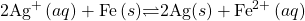## Solution

The reaction involves an oxidation-reduction reaction, so the standard cell potential can be calculated using the data in (Table 5.4.1).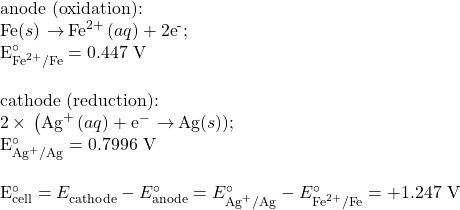With n = 2, the equilibrium constant is then: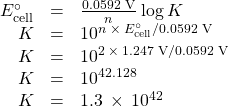The standard free energy is then: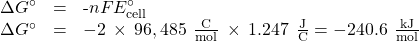The reaction is spontaneous, as indicated by a negative free energy change and a positive cell potential. The K value is very large, indicating the reaction proceeds to near completion to yield an equilibrium mixture containing mostly products.

What is the standard free energy change and the equilibrium constant for the following reaction at room temperature? Is the reaction spontaneous?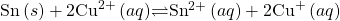### Solution

Spontaneous; n = 2;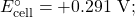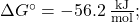K = 6.8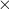109.

## Potentials at Nonstandard Conditions: The Nernst Equation

Most of the redox processes that interest science and society do not occur under standard state conditions, and so the potentials of these systems under nonstandard conditions are a property worthy of attention. Having established the relationship between potential and free energy change in this section, the previously discussed relation between free energy change and reaction mixture composition can be used for this purpose.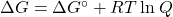Notice the reaction quotient, Q, appears in this equation, making the free energy change dependent upon the composition of the reaction mixture. Substituting the equation relating free energy change to cell potential yields the Nernst equation: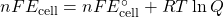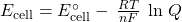This equation describes how the potential of a redox system (such as a galvanic cell) varies from its standard state value, specifically, showing it to be a function of the number of electrons transferred, n, the temperature, T, and the reaction mixture composition as reflected in Q. A convenient form of the Nernst equation for most work is one in which values for the fundamental constants (R and F) and a factor converting from natural to base-10 logarithms have been included: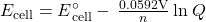Figure 5.5.2 – Predicting Redox Spontaneity Under Nonstandard Conditions

Use the Nernst equation to predict the spontaneity of the redox reaction shown below: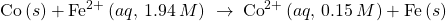## Solution

Collecting information from (Table 5.4.1) and the problem: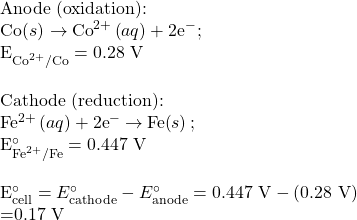Notice the negative value of the standard cell potential indicates the process is not spontaneous under standard conditions. Substitution of the Nernst equation terms for the nonstandard conditions yields: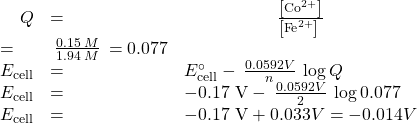The cell potential remains negative (slightly) under the specified conditions, and so the reaction remains nonspontaneous.

For the cell schematic below, identify values for n and Q, and calculate the cell potential, Ecell.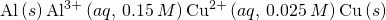n = 6; Q = 1440; Ecell = +1.97 V, spontaneous.

A concentration cell is constructed by connecting two nearly identical half-cells, each based on the same half-reaction and using the same electrode, varying only in the concentration of one redox species. The potential of a concentration cell, therefore, is determined only by the difference in concentration of the chosen redox species. The example problem below illustrates the use of the Nernst equation in calculations involving concentration cells.

Activity 5.5.3 – Concentration Cells

What is the cell potential of the concentration cell described by:

Zn(s) | Zn^{2+} (aq, 0.10 M)\|Zn^{2+}(aq, 0.50 M)|Zn(s)

## Solution

From the information given:

Anode:

Zn(s)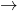Zn2+(aq, 0.10 M) + 2e

E°anode = -0.7618 V

Cathode:

Zn2+(aq, 0.50 M) + 2eZn(s)

E°cathode = -0.7618V

Overall:

Zn2+(aq, 0.50 M)Zn2+(aq, 0.10M)

E°cell = 0.000V

Substituting into the Nernst equation:

Ecell = 0.000V – (0.0592V)/2log (0.10/0.50) = +0.021V

The positive value for cell potential indicates the overall cell reaction (see above) is spontaneous. This spontaneous reaction is one in which the zinc ion concentration in the cathode falls (it is reduced to elemental zinc) while that in the anode rises (it is produced by oxidation of the zinc anode). A greater driving force for zinc reduction is present in the cathode, where the zinc(II) ion concentration is greater (Ecathode > Eanode).

The concentration cell above was allowed to operate until the cell reaction reached equilibrium. What are the cell potential and the concentrations of zinc(II) in each half-cell for the cell now?

Ecell = 0.000V; Zn2+cathode = Zn2+anode = 0.30M

# Key Concepts and Summary

Potential is a thermodynamic quantity reflecting the intrinsic driving force of a redox process, and it is directly related to the free energy change and equilibrium constant for the process. For redox processes taking place in electrochemical cells, the maximum (electrical) work done by the system is easily computed from the cell potential and the reaction stoichiometry and is equal to the free energy change for the process. The equilibrium constant for a redox reaction is logarithmically related to the reaction’s cell potential, with larger (more positive) potentials indicating reactions with greater driving force that equilibrate when the reaction has proceeded far towards completion (large value of K). Finally, the potential of a redox process varies with the composition of the reaction mixture, being related to the reactions standard potential and the value of its reaction quotient, Q, as described by the Nernst equation.

## Key Equations

•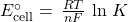•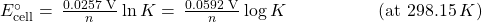•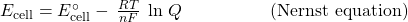•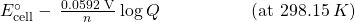• ΔG = −nFEcell
•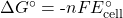•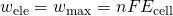End of Chapter Exercises

For each pair of standard cell potential and electron stoichiometry values below, calculate a corresponding standard free energy change (kJ).

(1a) 0.000 V, n = 2

(1b) +0.434 V, n = 2

(1c) −2.439 V, n = 1

Solution

(a) 0 kJ/mol; (b) −83.7 kJ/mol; (c) +235.3 kJ/mol

For each pair of standard free energy change and electron stoichiometry values below, calculate a corresponding standard cell potential.

(2a) 12 kJ/mol, n = 3

(2b) −45 kJ/mol, n = 1

Determine the standard cell potential and the cell potential under the stated conditions for the electrochemical reactions described here. State whether each is spontaneous or nonspontaneous under each set of conditions at 298.15 K.

(3a)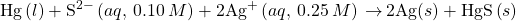(3b) The cell made from an anode half-cell consisting of an aluminum electrode in 0.015 M aluminum nitrate solution and a cathode half-cell consisting of a nickel electrode in 0.25 M nickel(II) nitrate solution.

(3c) The cell made of a half-cell in which 1.0 M aqueous bromide is oxidized to 0.11 M bromine ion and a half-cell in which aluminum ion at 0.023 M is reduced to aluminum metal.

Solution

(a) standard cell potential: 1.50 V, spontaneous; cell potential under stated conditions: 1.43 V, spontaneous; (b) standard cell potential: 1.405 V, spontaneous; cell potential under stated conditions: 1.423 V, spontaneous; (c) standard cell potential: −2.749 V, nonspontaneous; cell potential under stated conditions: −2.757 V, nonspontaneous

(4) Determine ΔG and ΔG° for each of the reactions in the previous problem.

Use the data in (Table 5.4.1) to calculate equilibrium constants for the following reactions. Assume 298.15 K if no temperature is given.

(5a)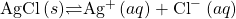(5b)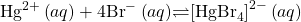Solution

(a) 1.710−10;  (b) 8.91019;

## Glossary

galvanic cell comprising half-cells of identical composition but for the concentration of one redox reactant or product
charge on 1 mol of electrons; F = 96,485 C/mol e
relating the potential of a redox system to its composition